Difference between revisions of "Gay-Berne model"

The Gay-Berne model is used extensively in simulations of liquid crystalline systems. The Gay-Berne model is an anistropic form of the Lennard-Jones 12:6 potential.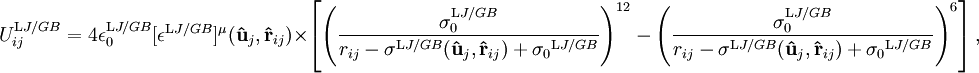$U_{ij}^{\mathrm LJ/GB} = 4 \epsilon_0^{\mathrm LJ/GB} [\epsilon^{\mathrm LJ/GB}]^{\mu} ( {\mathbf {\hat u}}_j , {\mathbf {\hat r}}_{ij} ) \times \left[ \left( \frac{\sigma_0^{\mathrm LJ/GB} } { r_{ij} - \sigma^{\mathrm LJ/GB} ({\mathbf {\hat{u}}}_j, {\mathbf {\hat{r}}}_{ij} ) + {\sigma_0}^{\mathrm LJ/GB} } \right)^{12} - \left( \frac { \sigma_0^{\mathrm LJ/GB} } { r_{ij} - \sigma^{\mathrm LJ/GB} ({\mathbf {\hat{u}}}_j, {\mathbf {\hat{r}}}_{ij} ) + {\sigma_0}^{\mathrm LJ/GB} } \right)^{6} \right],$ where, in the limit of one of the particles being spherical, gives: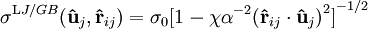$\sigma^{\mathrm LJ/GB} ({\mathbf {\hat{u}}}_j, {\mathbf {\hat{r}}}_{ij} ) ={\sigma_0}{[1 - \chi \alpha^{-2} {({\mathbf {\hat{r}}}_{ij} \cdot {\mathbf {\hat{u}}}_j )}^{2}]}^{-1/2}$

and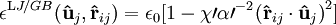$\epsilon^{\mathrm LJ/GB}({\mathbf {\hat{u}}}_j, {\mathbf {\hat{r}}}_{ij} ) ={\epsilon_0}{[1 - \chi\prime \alpha\prime^{-2} {({\mathbf {\hat{r}}}_{ij} \cdot {\mathbf {\hat{u}}}_j )}^{2}]}$

with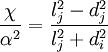$\frac{\chi}{\alpha^{2}}=\frac{l_{j}^{2}-d_{j}^{2}}{l_{j}^{2}+d_{i}^{2}}$

and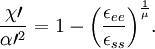$\frac{\chi \prime }{\alpha \prime^{2}}=1- {\left(\frac{\epsilon_{ee}}{\epsilon_{ss}}\right)} ^{\frac{1}{\mu}}.$